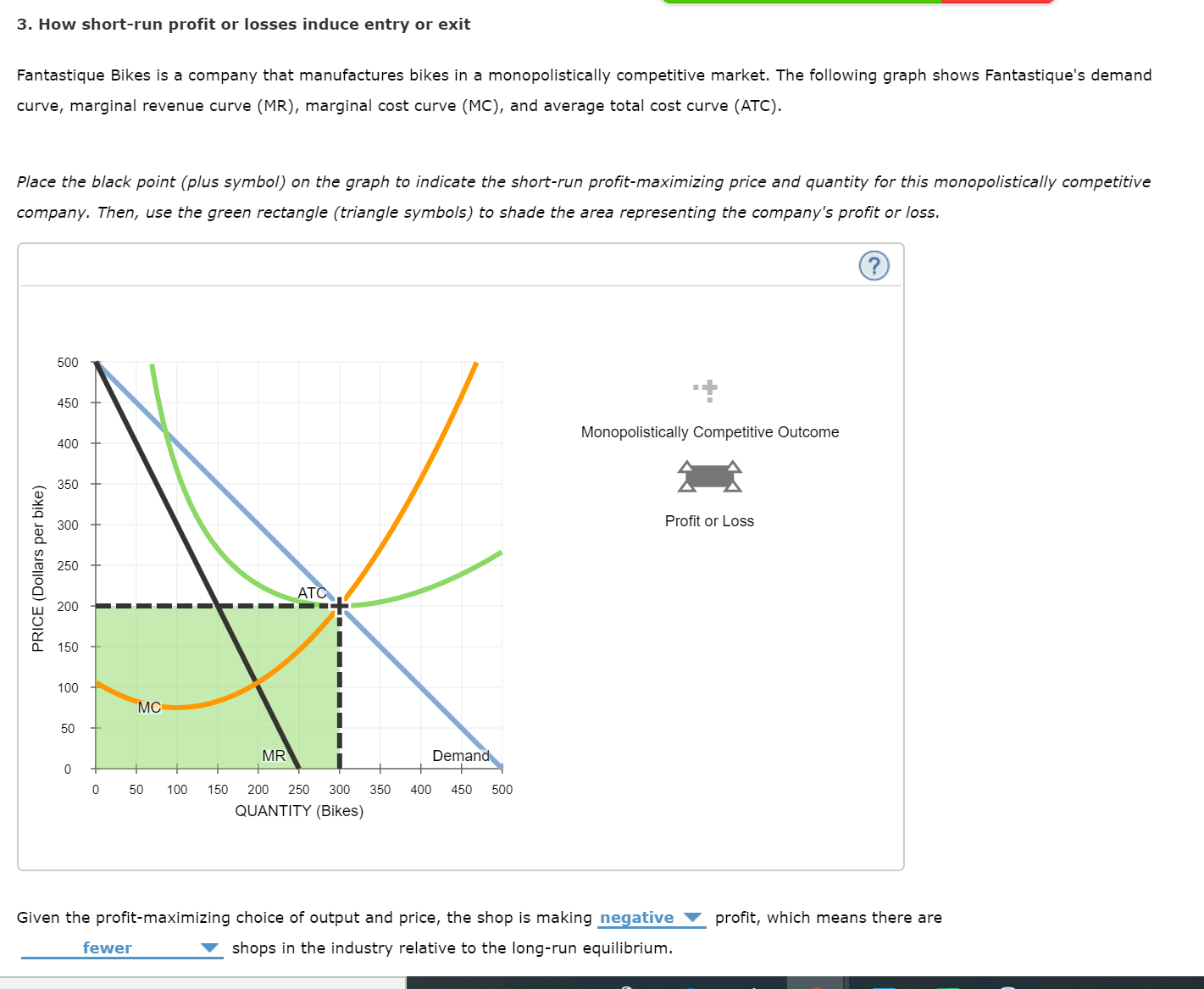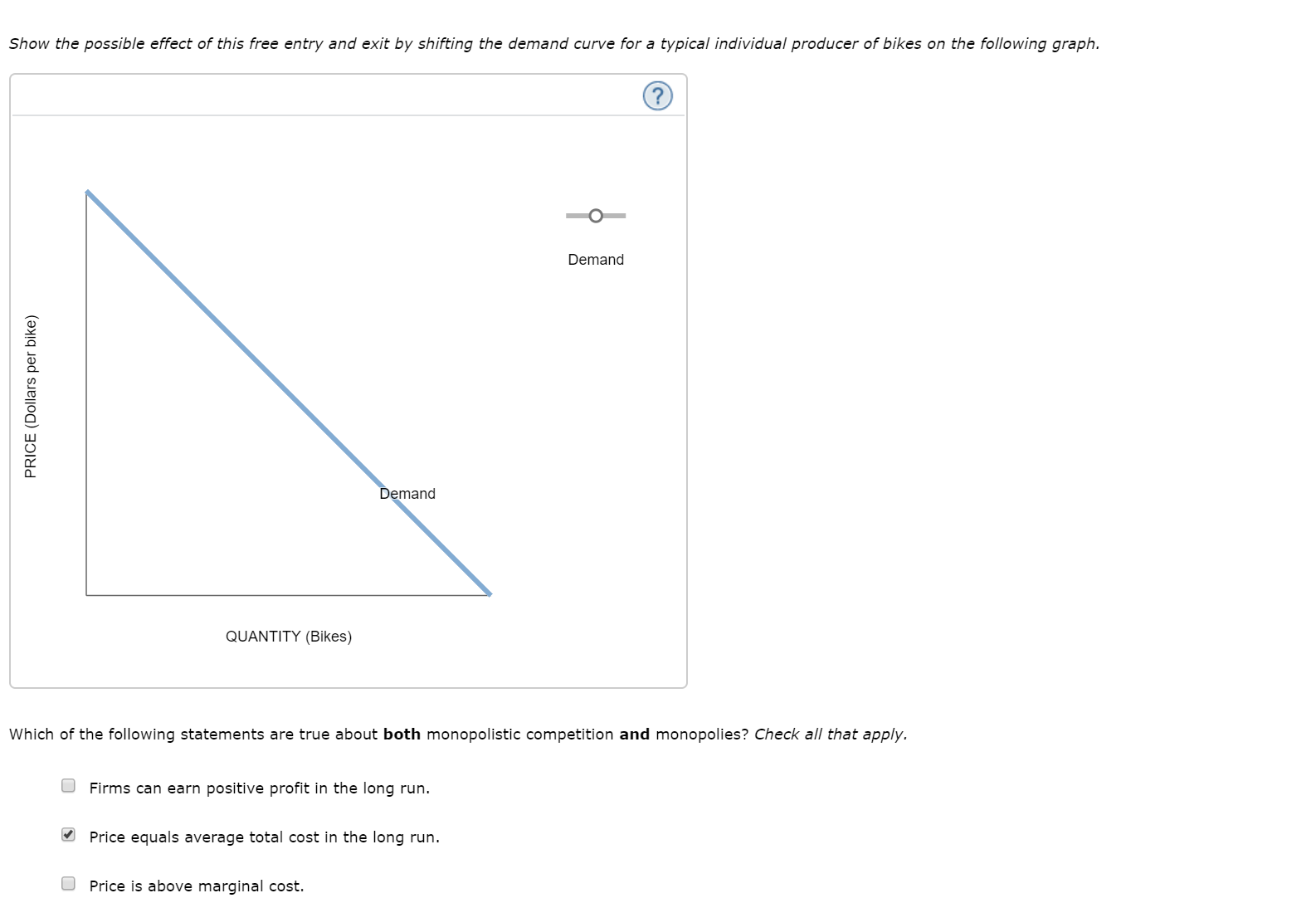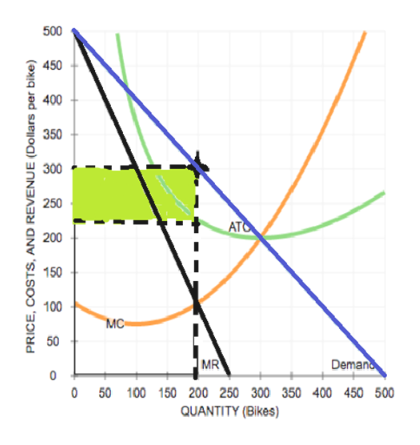# 3. How short-run profit or losses induce entry or exitFantastique Bikes is a company that manufactures bikes in a monopolistically competitive market. The following graph shows Fantastique's demandcurve, marginal revenue curve (MR), marginal cost curve (MC), and average total cost curve (ATC).Place the black point (plus symbol) on the graph to indicate the short-run profit-maximizing price and quantity for this monopolistically competitivecompany. Then, use the green rectangle (triangle symbols) to shade the area representing the company's profit or loss.500450Monopolistically Competitive Outcome400350Profit or Loss300250ATC200150100MC50MRDemand00100150200250300350400450500QUANTITY (Bikes)Given the profit-maximizing choice of output and price, the shop is making negativeprofit, which means there arefewershops in the industry relative to the long-run equilibrium.PRICE (Dollars per bike)50 Show the possible effect of this free entry and exit by shifting the demand curve for a typical individual producer of bikes on the following graph.DemandDemandQUANTITY (Bikes)Which of the following statements are true about both monopolistic competition and monopolies? Check all that apply.Firms can earn positive profit in the long runPrice equals average total cost in the long run.Price is above marginal cost.PRICE (Dollars per bike)

Questionhelp_outlineImage Transcriptionclose3. How short-run profit or losses induce entry or exit Fantastique Bikes is a company that manufactures bikes in a monopolistically competitive market. The following graph shows Fantastique's demand curve, marginal revenue curve (MR), marginal cost curve (MC), and average total cost curve (ATC). Place the black point (plus symbol) on the graph to indicate the short-run profit-maximizing price and quantity for this monopolistically competitive company. Then, use the green rectangle (triangle symbols) to shade the area representing the company's profit or loss. 500 450 Monopolistically Competitive Outcome 400 350 Profit or Loss 300 250 ATC 200 150 100 MC 50 MR Demand 0 0 100 150 200 250 300 350 400 450 500 QUANTITY (Bikes) Given the profit-maximizing choice of output and price, the shop is making negative profit, which means there are fewer shops in the industry relative to the long-run equilibrium. PRICE (Dollars per bike) 50 fullscreenhelp_outlineImage TranscriptioncloseShow the possible effect of this free entry and exit by shifting the demand curve for a typical individual producer of bikes on the following graph. Demand Demand QUANTITY (Bikes) Which of the following statements are true about both monopolistic competition and monopolies? Check all that apply. Firms can earn positive profit in the long run Price equals average total cost in the long run. Price is above marginal cost. PRICE (Dollars per bike) fullscreen
check_circle

Step 1

The demand curve, marginal revenue curve as well as the marginal and average cost curves of the firm is given in the figure. The monopolistically competitive firm maximizes its profit at the point where the marginal revenue equals the marginal cost. This is the profit maximizing output of the monopolistically competitive firm. The MR equals MC for the firm at the point of 200 bikes. This profit maximizing quantity when extended to intersect with the demand curve gives the profit maximizing price of the firm which is obtained at the price of \$300. From the diagram, the average total cost of producing a bike is intersecting the profit maximizing output at the price of \$225. Thus, the profit of the firm is found to be \$75 per unit of bike sold. Thus, it can be illustrated as follows:help_outlineImage Transcriptionclose500 450 400 350 300 250 ATO 200 150 100 MC 50 Deman MR 0 50 100 150 200 250 300 350 400 450 500 QUANTITY (Bikes) PRICE, COSTS, AND REVENUE (Dollars per bike) fullscreen
Step 2

From the profit maximizing point of the firm, it is identified that there is an economic profit of \$75 per bike sold by the firm. the presence of the economic profit in the short run indicates that there will be fewer competitors in the market compared to the long run equilibrium because, under the long run, more new firms would enter into the market leading to the increased supply and eliminating the presence of economic profit. Thus, the firm earns positive economic profit which means there are fewer shops in the industry relative to the long run equilibrium.

Step 3

In the long run, the presence of the economic profit for a typical firm attracts the new firms into the market. This increases the number of competitors in the market. When the number of the competitors increases, the demand for the typical firm would decline ...

### Want to see the full answer?

See Solution

#### Want to see this answer and more?

Solutions are written by subject experts who are available 24/7. Questions are typically answered within 1 hour.*

See Solution
*Response times may vary by subject and question.
Tagged in